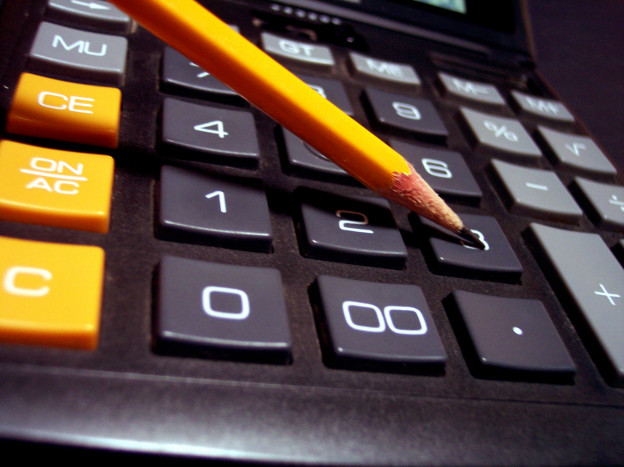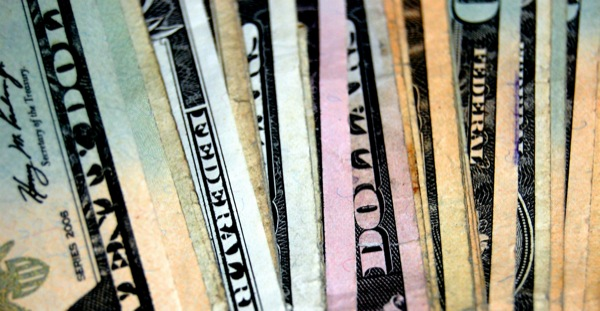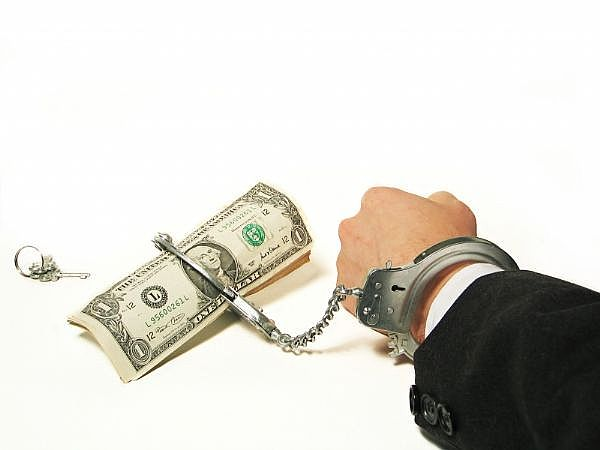###### Save. Spend. Splurge.# Learn some basic math and save a lot of money! (PLEASE…!!)

People listen up!!

## The majority of you out there suck at basic math.

I am not even talking about math that you (rightfully) thought was utterly useless for real life everyday situations such as geometry or calculus, but about basic, everyday math that even a 5th grader could do.

I am not even asking that you do this basic, elementary math with a pencil and piece of paper — I’m even letting you use a calculator (something you ALL HAVE on your fancy smartphones or what have yous).

## But you suck at basic math and need to learn how to do it, because it is costing you MONEY in stores.

Actual. Money.

*RAARRRRRRGH!!!*## RETAILERS USE NUMBERS TO SCREW WITH YOU

I’d highly recommend reading Priceless: The Myth of Fair Value (and How to Take Advantage of It, along with this post I wrote with J. Crew as an example of what “anchor pricing” was.

If you want more material to read, the proof is in the pudding.

Specifically this pudding.

You walk into a Starbucks and see two deals for a cup of coffee.

The first deal offers 33% extra coffee.

The second takes 33% off the regular price.

What’s the better deal?

[…]

The deals appear to be equivalent, but in fact, a 33% discount is the same as a 50 percent increase in quantity.

Math time: Let’s say the standard coffee is \$1 for 3 quarts (\$0.33 per quart).

The first deal gets you 4 quarts for \$1 (\$0.25 per quart) and the second gets you 3 quarts for 66 cents (\$.22 per quart).

The upshot: Getting something extra “for free” feels better than getting the same for less.

Did that example confuse you?

It shouldn’t have.

Have a chai latte and read on.

## REAL LIFE SCENARIO: \$180 DOWN THE DRAIN IN A MATTER OF SECONDS

Just the other day, when bought our cutlery on sale at an additional 25% off (FINALLY! After 2 years of eying sales and choosing the best time to buy them), but unfortunately they did not have all the sets we needed in stock.

We were apologetically told that it was because a woman came in and bought 12 sets that morning, and they would have to special order them in for us, but would ring it up today and pay for it, then we could pick up the sets another day.

Fine. No problem.

As we watched her ring up the amounts, we realized that she had overcharged us by about \$15 per set.

## \$15 x 8 sets = \$120 saved!

When we pointed out the mistake, she tried to argue with us that it was THAT PRICE in the system, until we brought her the ticket to show her that they had forgotten to change the price.

She whisked the ticket away, and honoured the “old” price of the sets, which I suppose in 4 months had gone up by \$15 a set.

As the hamsters churned in my head, happily relieved that we would not be paying that \$15 premium per set, I realized that the woman who came by this morning and bought 12 of the exact same sets didn’t even bother to check.

Why would she?

She either just assumed it was going to be the price that was displayed & didn’t bother to check her receipt or couldn’t do basic math to save her life because there was an additional 25% off and she didn’t want to bother figuring out if she had been charged correctly.

## \$15 x 12 sets = \$180 down the drain

Yep.

She paid almost \$200 more (before taxes) on something in a matter of seconds.

(Of course this ended up being good news for us, seeing as the tickets would have been changed by the time we came by for the sets, which meant we would have paid \$120 more.)

Basic math is also useful in figuring out how a tiered tax system works. It’s not just for shopping but you can exercise your grey matter when you shop so that you become a smarter shopper.

## WHAT ARE SOME BASIC MATH SKILLS I THINK YOU SHOULD MASTER?

Other than solving that coffee problem above?

The first deal offers 33% extra coffee.

The second takes 33% off the regular price.

What’s the better deal?

1. How to structure a math problem
2. How to calculate a percentage
3. How to calculate an average
4. How to calculate a discount in dollar amounts
5. How to calculate tiered percentages (e.g. like our taxes)
6. How to convert something (e.g. kilograms to pounds, knowing the conversion ahead of time)

If you want to test yourself and/or are bored, read on for some math problems.

## If you are familiar with math, each problem should only take you less than a minute or 60 seconds to solve with Excel.

Longer if you are using pen and paper or a calculator.## PROBLEM #1: STRUCTURING A MATH PROBLEM (WITH PERCENTAGES)

Let me use my cutlery example above and give you the facts:

• Each set of cutlery was \$74.95
• If you bought 4 sets, the package price was \$249.95
• On top of the sets of 4, was another discount of 25%
• Taxes are 13%
• We wanted to buy 8 sets of cutlery

Solve the following:

1. How much we would have paid, had we purchased each set of cutlery separately without the package price, but with the 25% off after taxes?
2. How much did we actually pay with the package price, and with 25% off, after taxes?

.

.

.

.

.

.

.

.

.

.

# \$508.16

This is how you should structure the problem (in order):

Each set separately as a base price without the combined package price of buying a set of 4:

\$74.95 x 8 = \$599.60

With the 25% discount:

\$599.60 x 0.75 = \$449.70

(I put 0.75 as the multiplier because 25% is represented as 0.25 and since it is a discount, I want to know the rest of the price not included which would be 1 – 0.25 = 0.75)

Now with 13% taxes after the 25% discount:

\$449.70 x 1.13 = \$508.16

# \$423.67

This is how you should structure the problem (in order):

Two package sets of 4 (equivalent to 8 individual sets as above) with the package price:

\$249.95 x 2 = \$499.90

With the 25% discount:

\$499.90 x 0.75 = \$374.93

Now after taxes of 13%:

\$374.93 x 1.13 = \$423.67## PROBLEM #2: HOW TO CALCULATE AN AVERAGE DISCOUNT

Buy one item at \$50 get the second one of \$50 at 50% off.

Solve the following:

1. What’s your average percentage discount?
2. What’s your total dollar discount?
3. What if the first item cost \$75 and the second was \$55.85? What would be your dollar discount and subsequent percentage discount?

.

.

.

.

.

.

.

.

.

.

## 1. What’s your average percentage discount?

Two items with the same \$50 dollar amount, with the discount split between the two

50% / 2 = 25% off

## 2. What’s your total dollar discount?

Second item is 50% off

\$50 * 0.50 = \$25

## What would be your dollar discount and average percentage discount on both items?

Find the base total of the items before discounts

\$75 + \$55.85 = \$130.85

Find the 50% off discount that applies to the second item only

\$55.85 x 0.50 = \$27.93

Your total dollar discount is \$27.93.

Find the subsequent average percentage discount on both items

\$27.93 / \$130.85 = 0.213

0.213 x 100 = 21.34%

Your average percentage discount is 21.34%.## PROBLEM #3: HOW TO CALCULATE TIERED TAXES

• 15% on the first \$43,561 of taxable income, +
• 22% on the next \$43,562 of taxable income (on the portion of taxable income over \$43,561 up to \$87,123)
• 26% on the next \$47,931 of taxable income (on the portion of taxable income over \$87,123 up to \$135,054)

Solve the following:

1. How much do you pay in taxes if you earn \$44,500?

.

.

.

.

.

.

.

.

.

.

## 1. How much do you pay in taxes if you earn \$44,500?

Find out where the tax rate changes

In this case the tax rate would increase to 22% on any amount above \$43,561.

Your total salary falls a little over this tax rate increase of 22% but under the 26% bracket increase.

Calculate the first tax rate

0.15 x \$43,561 = \$6534.15

Calculate the dollar difference between \$43,561 and your salary of \$44,500 (for the next income tax bracket)

\$44,500 – \$43,561 = \$939

Calculate the second tax rate on the \$939

0.22 x \$939 = \$206.58

Add the two tax rates together

\$6534.15 + \$206.58 = \$6740.73

Your total tax payable is: \$6740.73

## PROBLEM #4: HOW TO CALCULATE CONVERSIONS

It costs \$4.29 per kilogram for some fudge.

The conversion of kilograms to pounds is roughly: 1 kg = 2.20 lbs

(Seriously it’s so effed up here in Canada that supermarkets charge in kilograms but show the price in pounds because it looks cheaper.

I have to do this calculation all the time, or memorize the little kilogram price by squinting at the ticket.)

Solve the following:

1. How much would it cost for 1 pound of fudge (even though you know you have no willpower and you’d probably buy 5 pounds)?
2. Okay you caved. You bought 5 pounds of fudge because you’re a fudge whore. How much would you have to pay if it was strictly buy 3 pounds of fudge, and get the 4th at 33% off?

.

.

.

.

.

.

.

.

.

.

## 1. How much would it cost for 1 pound of fudge?

Get the cost per pound using the kilogram price and the conversion

\$4.29 / 2.20 = \$1.95

It is \$1.95 per pound.

## How much would you have to pay if it was buy 4 pounds of fudge, but only get the 4th pound at 33% off?

Get the cost per pound using the kilogram price and the conversion

\$4.29 / 2.20 = \$1.95

It is \$1.95 per pound.

3 pounds of fudge at full price (100%)

3 x \$1.95 = \$5.85

4th one is 33% off

\$1.95 x 0.67 = \$1.30

5th one at full price (100%)

\$1.95 x 100% = 1.95

\$5.85 + \$1.30 + \$1.95 = \$9.10

## 3. What are the savings in dollar amounts and percentages?

Find the original price with no discounts whatsoever

5 x \$1.95 = \$9.75

Find the dollar savings

\$9.75 – \$9.10 = \$0.65

You saved \$0.65 in total.

Calculate the dollar savings over the original price

\$0.65 / \$9.75 = 0.0667

0.0667 x 100 = 6.67%

You saved 6.67% on fudge, which totally justifies eating 5 pounds all by yourself and not letting your little crumb crunching toddler have any.

## IF YOU CAN’T DO THESE PROBLEMS ABOVE, YOU NEED A BRUSH UP IN BASIC ELEMENTARY MATH.

You could be losing a lot of money otherwise, especially if you let other people do the math for you and just assume it’s correct.

•##### Olivia

Just saw this post via instagram – thanks!!

•##### Sherry of Save. Spend. Splurge.

Thank you for coming from IG!

•##### Dean

•